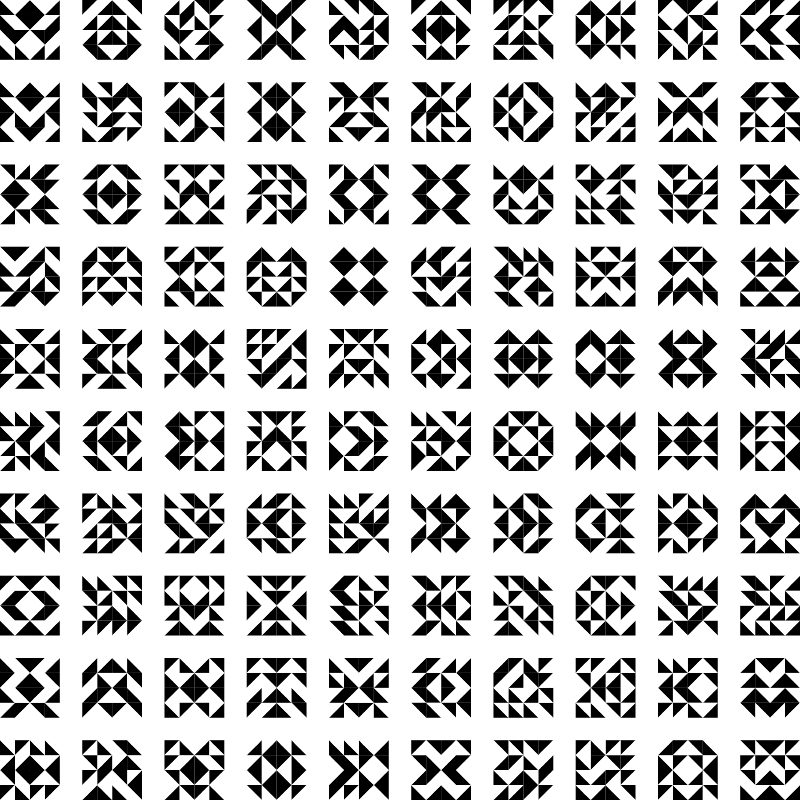Steven H. Cullinane’s Diamond TheoryAuthor: Alexis Praga
> import Diagrams.Backend.SVG.CmdLine

We recreate the “diamond theory” by Steven H. Cullinane (see the Recode project http://recodeproject.com/artwork/v2n1diamond-theory).

> {-# LANGUAGE NoMonomorphismRestriction #-}
>
> import System.Random
> import Data.List.Split

The idea is to generate a matrix of tiles, where each tile is a square with random black and white right triangles in it triangle. In practice, each tile is composed of 4 smaller (square) tiles and each of them has 4 very small tiles. We note the tiles “large”, “medium” and “small” respectively in the code.

We first define the small tile. It is composed of a square split into two right triangles. The upper left triangle is always white, the lower right triangle is always black.

> side = sqrt 2
> triangleRect :: Diagram B
> triangleRect = polygon ( with
>   & polyType .~ PolySides
>      [ 135 @@ deg, 90 @@ deg]
>      [ 1        , side      ]
>   )

When defining each triangle, the enveloppe do not take the linewidth (lw) into account so we set it to none. This will cause issues later on.

> triangleLeft = triangleRect # rotateBy (1/2) # fc white # lc white # lw none
>
> triangleRight = triangleRect # fc black #lc black # lw none

For the small tile, we enforce the old behaviour for the origin of the tile as we want the triangles to be composed at the point of tangency, enforced by “align”.

> smallTile = beside (r2 (1,-1)) (triangleLeft # align (r2 (1, -1)))
>                                 triangleRight

For interesting results, the small tiles are rotated randomly with angles in \{0, \frac{\pi}{2}, \pi, \frac{3 \pi}{2} \}.

> smallTile' :: Int -> Diagram B
> smallTile' x = smallTile # rotate x'
>   where x' = fromIntegral x *pi/2 @@ rad

Now we can create the medium tile, where 4 small tiles are placed in a matrix-like fashion. The origin must be placed at the center with align

> createMatrix x = matrix # alignX 0 # alignY 0
>   where matrix = (x !! 0 ||| x !! 1 )
>                          ===
>                  (x !! 2 ||| x !! 3)
>
> mediumTile angles = createMatrix (map smallTile' angles)

We then create the large tiles as a composition of 4 medium tiles. For even more interesting results, we use a random number of axis of symmetry (between 0 and 2). Here, we take list of 16 angles as input, where each angle corresponds to a random rotation for the small tiles. Beware reflectX is actually a reflection in respect to the Y axis, so the naming convention is inverted.

> largeTile :: [Int] -> Bool -> Bool -> Diagram B
> largeTile angles xSymmetry ySymmetry = createMatrix [a, b, c, d]
>   where
>     a = mediumTile $chunks !! 0 > b = if ySymmetry then a # reflectX else mediumTile$ chunks !! 1
>     c = if xSymmetry then a # reflectY else mediumTile $chunks !! 2 > d > | ySymmetry && xSymmetry = a # rotateBy (-1/2) > | ySymmetry = c # reflectX > | xSymmetry = b # reflectY > | otherwise = mediumTile$ chunks !! 3
>     chunks = chunksOf 4 angles
>
> -- Needs a list of 16 angles and the number of axes
> largeTile' :: ([Int], Int) -> Diagram B
> largeTile' x = largeTile n xSymmetry ySymmetry
>   where
>     n = fst x
>     nbAxes = snd x
>     xSymmetry = nbAxes == 1 || nbAxes == 3
>     ySymmetry = nbAxes == 2 || nbAxes == 3

Finally, we create an array of large tiles by using position. The random angles and number of axis of symmetries are generated here, at the higher level. This allows us to only generate two random list. However, they must be split into chunks accordingly. As a final note, the bug with the linewidth will most likely appear in the final results as very fine gapes between the small tiles.

> centerPos x = (x-0.5)*4 + (x-1)*d
>     where d = 1.5
>
> randInts :: Int -> [Int]
> randInts seed = randomRs (0, 3) (mkStdGen seed)
>
> example :: Diagram B
> example = position (zip (map p2 pos) (zipWith (curry largeTile') angles nbAxes))
>   where
>     nb = 10
>     pos = [(centerPos x, centerPos y) | x <- [1..nb], y <- [1..nb]]
>     angles = take (nb*nb) $chunksOf 16$ randInts 15
>     nbAxes = take (nb*nb) \$ randInts 12
> main = mainWith (example :: Diagram B)